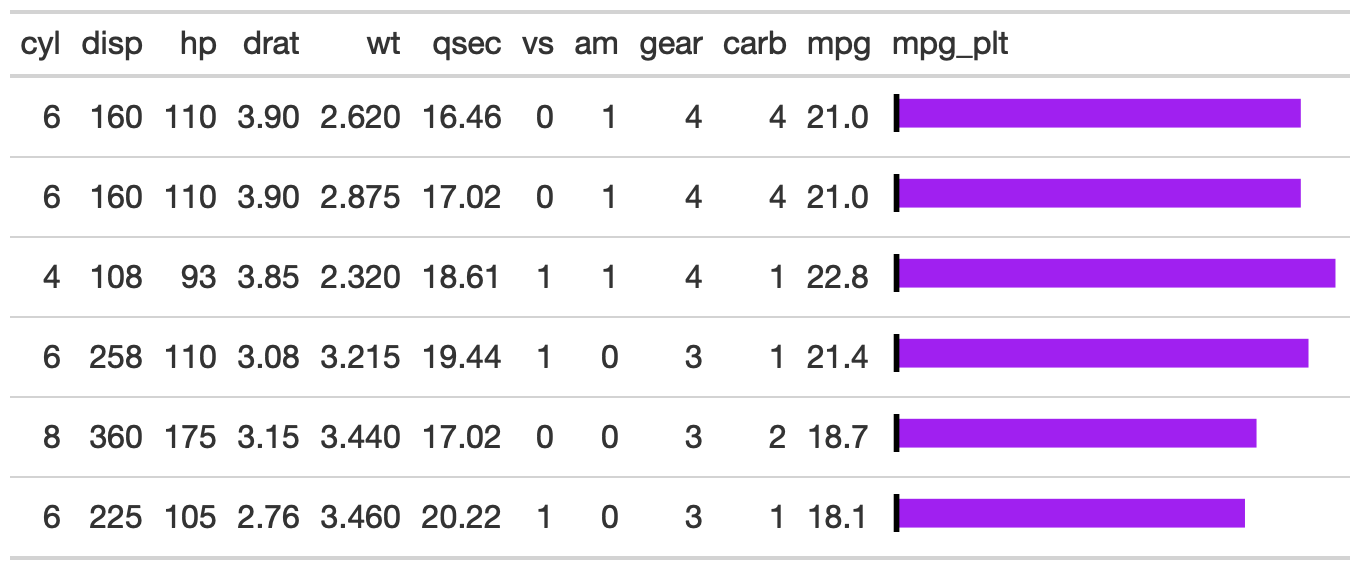The gt_plt_bar function takes an existing gt_tbl object and adds horizontal barplots via ggplot2. Note that values are plotted on a shared x-axis, and a vertical black bar is added at x = zero.

## Usage

gt_plt_bar(
gt_object,
column = NULL,
color = "purple",
...,
keep_column = FALSE,
width = 70,
scale_type = "none",
text_color = "white"
)

## Arguments

gt_object

An existing gt table object of class gt_tbl

column

A single column wherein the bar plot should replace existing data.

color

A character representing the color for the bar, defaults to purple. Accepts a named color (eg 'purple') or a hex color.

...

Additional arguments passed to scales::label_number() or scales::label_percent(), depending on what was specified in scale_type

keep_column

TRUE/FALSE logical indicating if you want to keep a copy of the "plotted" column as raw values next to the plot itself..

width

An integer indicating the width of the plot in pixels.

scale_type

A string indicating additional text formatting and the addition of numeric labels to the plotted bars if not 'none'. If 'none', no numbers will be added to the bar, but if "number" or "percent" are used, then the numbers in the plotted column will be added as a bar-label and formatted according to scales::label_percent() or scales::label_number().

text_color

A string indicating the color of text if scale_type is used. Defaults to "white"

## Value

An object of class gt_tbl.

## Examples

library(gt)
gt_plt_bar_tab <- mtcars %>%
gt_plt_bar(column = mpg, keep_column = TRUE)Other Plotting: gt_plt_bar_pct(), gt_plt_bar_stack(), gt_plt_dist(), gt_plt_percentile(), gt_plt_point(), gt_plt_sparkline(), gt_plt_winloss()